## States of Matter

In the States of Matter, introducing Matter is:

• Everything in our surroundings is called matter. They may be the food we eat, the vehicles, the gadgets, the day-to-day materials that we use, the air we breathe or the water that we drink.
• These things occupy some space and have mass and volume.
• For instance, in classrooms the benches that the students use to sit occupy some space of the classroom. They have mass and volume, and hence they can be regarded as matter.
• According to the Indian philosophers, the matter can be classified into five primitive elements.
We also know them as Pancha Tatva:• air, sky, soil, fire, and water.
• Every living or non-living is made up of these five primitive elements.
• Every state of matter has its own properties.

## There are 5 States of Matter :

• Solid
• Liquid
• gas
• Plasma
• BEC (Bose-Einstein condensate).

### Solid:They have definite shape due to strong intermolecular forces of attraction.
• & distinct boundaries.
•  fixed volume
• cannot flow.
• negligible compressibility due to negligible distance between the neighbouring molecules.
• possess a tendency to uphold their shape when exposed to an external force.
•  break under force but it is difficult to change their shape so they are rigid.
•  high density and do not diffuse at all

### Liquid:

• A liquid has no definite shape and takes up the shape of the container in which it is kept.
•  but definite volume due to weaker intermolecular force of attraction than solids.
• They can flow from a higher level to a lower level.
•  It is compressible due to larger distance between the neighboring molecules than solid but lesser than gas.
• They have a lower density.

A liquid can diffuse into another liquid due to the fact that molecules move faster in a liquid but is slower as compared to gases.

### Gas:

• They do not have a definite shape and take up the shape of the container.
• & possess definite volume due to weakest intermolecular forces not rigid.
• Easily compressible due to excess space between the particles of gas which compresses on applying pressure.
• It can easily undergo diffusion due to the fact that molecules in a gas move at a very fast rate due to which speed of diffusion is very large.
•  They can flow in all possible directions.

### Plasma: • It is a state of matter similar to gas, in which a certain position of the gaseous particles is ionized. • Due to the average strength of the electrical forces. the plasma is neutral.• Commonly found in the universe plasma is naturally occurring in flames, lightning, auroras

BEC (Bose-Einstein condensate)

• It is a gaseous superfluid phase formed by atoms cooled to a temperature very near to absolute zero
• & can be thought of as the opposite of plasma.

## Interconversion of States of matter: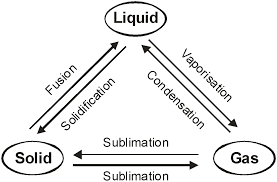•  Melting point: The temperature at which solid-state converts into a liquid state. It decreases in the presence of impurity.
•  Boiling point: The temperature at which vapour pressure of a liquid becomes equal to atmospheric pressure. It increases with increase in impurity and with rising in temperature.
• The boiling point of water is 100°c
• Freezing point: The temperature at which a liquid state converts into a solid-state.
• The freezing point of water is 0°c
• Evaporation: The process of conversion of liquid into vapours at any temperature.
• Due to evaporation:
• Water droplets appear on the outer surface of a glass containing ice-cold water.
•  Water kept in earthen pots becomes cool during summer.
Factors deciding physical states of substance:
• For gaseous state, a force of attraction << thermal energy.
• For liquid state, a force of attraction > thermal energy.
• For solid-state, a force of attraction >> thermal energy

### Intermolecular Forces in States of matter

• Intermolecular forces can be described as the forces of attraction and repulsion existing between the interacting particles of atoms and molecules in a compound
• They call the attractive intermolecular forces.
• van der Waals forces, which include dispersion forces or London forces, dipole-dipole forces, and dipole-induced dipole forces.
• Intermolecular forces in case of solids are very strong. The constituent particles are closely packed thereby making the solids incompressible and of high density.

1. Dispersion forces or London forces: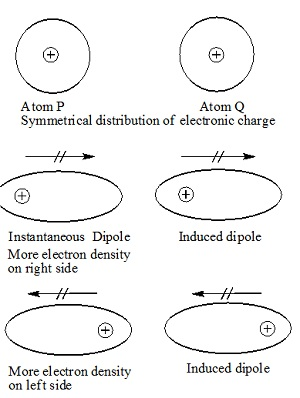Dispersion forces or London forces present between non- polar atoms and molecules, e.g among the atoms of chlorine molecules. They are the weakest intermolecular forces .there forces increases with

I. Increase in no of electrons in molecules,
II. Increase in molecular size

2. Dipole-dipole interaction :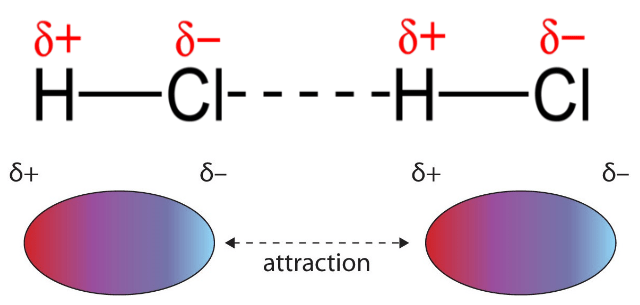Dipole-dipole interaction forces act between the molecules possessing permanent dipoles. The interaction is stronger than London forces and weaker than ion-ion interaction.

3. Dipole induced dipole forces:Dipole induced dipole forces to act between the polar molecules having a permanent dipole and the molecules lacking permanent dipoles.

4. Hydrogen bond :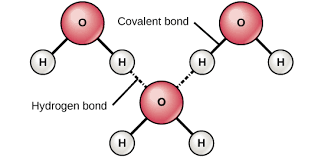The hydrogen bond is a special case of dipole-dipole interactions. It is found in molecules in which highly polar bonds are present N-H, O-H, Or H-F bonds are present.

5. Thermal Energy:
Thermal energy refers to the energy of a body that arises from the motion of its atoms or molecules.
The thermal energy of a substance is directly proportional to the temperature of the
Substance.

## The Gaseous State Of Matter:

• This is the simplest state of matter. Characteristics of this ‘state of matter are’
• In gases, the intermolecular forces are weakest.
• highly compressible.
• exert pressure equally in all directions.
• A much lower density than the solids and liquids.
•  The volume and the shape of gases are not fixed.
• Gases mix evenly and completely in all proportions with no mechanical aid.
• Measurable Properties of Gases:
Mass:
we express it in gram or kg.
Volume :
It is equal to express  the volume of the container in terms of litre (L), millilitre (mL), cubic centimetre (cm), cubic metre (m ) or cubic decimetre (dm ).
1 L = 1000 mL = 1000cm3 = 1dm3
• 1m3 = 103dm3 = 106 cm3 = 106 mL = 103 L
• Pressure:
Gas pressure is measured with manometer and atmospheric pressure is measured by barometer.
1 atm = 76 cm of Hg = 760 mm of Hg = 760 torr
1 atm = 101.325 kPa = 101325 Pa = 101.325 Nm-2
= 1.01325 bar
1 bar5 = 105 Pa. =0.987atm
Measurement of the pressure of gas:
•  Open-end manometer, pgas = patom – h
• Closed end manometer, pgas = h

Where,

h is the difference in the mercury levels in the two columns of density (d) (of a gas)
Temperature:
It is measured in celsius scale (°C) or in Kelvin scale (K).
SI unit of temperature is Kelvin (K), T (K) = t°C+ 273
Standard temperature and pressure (STP or NTP):
It means 273.15 K (O°C) temperature and1 bar (i.e., exactly 105 pascal) pressure.
At STP, the molar volume of an ideal gas is 22.71098 L mol-1

## Laws in States Of Matter (Gaseous State) :

### Boyle’s Law :

The volume of a given mass of a gas is inversely proportional to its pressure at a constant temperature.
V ∝ 1/ p or Vp = K

K is a constant and its value depends on the mass, temperature, and nature of the gas.
∴ p1V1 = p2V2
Isotherms: Graphs of V vs p or pV vs p at a constant temperature known as Isotherms.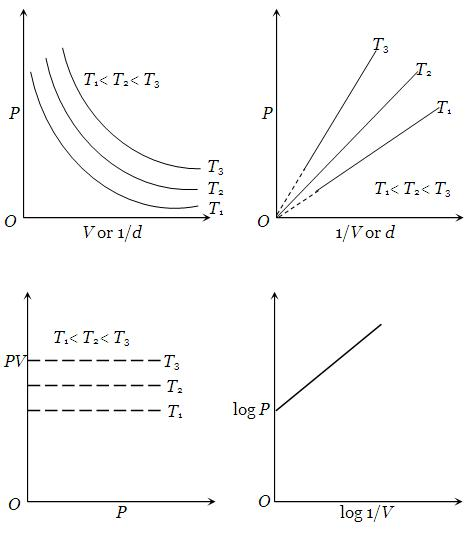### Charles’ Law:

The volume of the given mass of a gas increases or decrease by 1 / 273 of its volume for each degree rise or fall of temperature respectively at constant pressure
Vt = Vo (1 + t/273) t constant p
Or,
The volume of a given mass of a gas is directly proportional to the absolute temperature at constant pressure.
V ∝ T (at constant p), V / T = constant or
V1/T1 = V2/T2
Absolute zero is the theoretically possible temperature at which the volume of the gas becomes zero. It is equal to O°C or 273.15K.
Isobars: A graph of V vs T at constant pressure is known as isobar.### Gay Lussac’s Law:

The pressure of a given mass of gas increases or decreases by 1 /273 of its pressure for each degree rise or fall of temperature respectively at constant volume.
pt = po (1 + t / 273) at constant V and n
or
The pressure of a given mass of a gas at constant volume is directly proportional to absolute temperature.
p ∝ T or p = KT or p/T = K at constant V and n or
P1/T1 = P2/T2
Isochores: A graph of p vs T at constant volume is known as isochore.### Avogadro’s Law in Gaseous State Of Matter:

It states that equal volumes of all gases under the same conditions of temperature and pressure contain an equal number of molecules.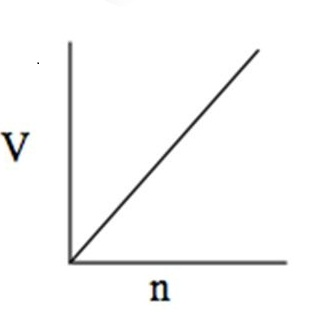Mathematically,

V ∝ n (at constant T and p)
or V/n =K
Molar gas volume: The volume of one mole of a gas, i.e., 22.4 L at STP (0°C, 1 atm) is known as molar gas volume.

## Ideal Gas Equation In States of Matter :

V ∝ 1/p, T and n constant (Boyle’s law)
V ∝ T, p and n constant (Charles’ law)
V ∝ n, p and T constant (Avogadro’s law)
V ∝ nT / p or pV ∝ nT
pV = nRT.
This is known as the ideal gas equation.

From the ideal gas equation,

Density:

D=PM/RT

### Numerical value of ‘R’

R is known as the universal gas constant.

The numerical value of ‘R’

= 0.0821 L atm mol-1 K-1
= 0.083 L bar mol-1 K-1
= 8.314 JK mol-1
=8.314 * 107 erg K-1 mol-1
= 1.987 or 2 cal K-1 mol-1

## Ideal gas:

The gas which obeys the equation pV = nRT at every temperature and pressure range strictly Is known as Ideal gas.

### Real gases:

Since none of the gases present in the universe strictly obey the equation pV =nRT. hence they are known as real or non-ideal gases.

Real gases behave, ideally at low p and high T.

## Graham’s Law of Diffusion in States of matter

• Under Similar conditions of temperature and pressure, the rates of diffusion of gases are inversely proportional to the square root of their densities.
Mathematically,
• r1/r2 = √d2/√d1 = √M2√M1
• If two gases are taken at different pressures, then, as greater the pressure, greater is the
a number of molecules hitting per unit area, greater is the rate of diffusion.
• R1/R2 = P1/P2 = √d2/√d1== P1/P2= √M2√M1
For two gases undergoing diffusion at the same pressure but at two different temperatures:
• R1/R2= √T1d2/T2d1=√T1M2/T2M1

Comparison of volumes of two different gases effused/diffused during the same time interval

• R1/R2=V1/t/V2/t = V1/V2 =√d2/√d1 ==√M2√M1
Where V1 is the volume of gas 1 diffused/effused in time t and V2 is the volume of gas 2
diffused/effused at the same time t under the same conditions of temperature and
pressure.
• Comparison of times taken for effusion/diffusion of the same volume of two different
Gases
• R1/R2=V/t1/Vt2 = t2/t1 = √d2/√d1 ==√M2√M1

Where,

t is the time taken for the gas 1 for effusion/diffusion of volume V and t2 is the
time is taken for gas 2 for effusion/diffusion of the same volume under the same conditions
[Diffusion is the tendency of gases to distribute itself uniformly throughout the available space while effusion is the movement of gas through a small hole when it is subjected to pressure].

### Dalton’s Law of Partial Pressure At constant temperature in States of matter

The total pressure exerted by a mixture of non-reacting gases is the sum of partial pressures of different gases present in the mixture.

p = p1 + p2 + p3 +…

The partial pressure of a gas = mole fraction of the gas * total pressure. If n1, n2, and n3 are moles of non-reacting gases filled in a vessel of volume V at temperature T, the total pressure, p is given by

pV = (n1+ n2 + n3 )RT

This is the equation of state of a gaseous mixture,

[Aqueous tension: It is the pressure exerted by water vapours at a particular temperature. It depends upon temperature.] • The pressure of a dry gas can be determined by Dalton’s law. When a gas is collected over water, its observed pressure is equal to the sum of the pressure of dry gas and the pressure of water vapour (aqueous tension) then
The pressure of dry gas = pressure of moist gas – aqueous tension.

## Kinetic Theory of Gases related to States of matter :

• Main assumptions of this theory are:
•  A gas consists of a large number of small particles, called molecules.
•  The volume occupied by gas molecules is negligible as compared to the total volume of the gas.
• There is a continuous rapid random motion of gas molecules. The molecules collide with each other and with the walls of a container.
• The molecules are perfectly elastic bodies and there is no loss of kinetic energy during collisions.
•  There are no attractive forces between the gaseous molecules.
•  The pressure exerted by a gas is due to the bombardment of gas molecules against the walls of the container.
• The different molecules possess different velocities and hence different energies. The average KE is directly proportional to absolute temperature.
KE =3/2 RT
Average K.E per molecule = 3/2KT   here,

K= Boltzman constant: It is gas constant per molecule
k = R/NA = 1.38*10-23 JK-1 mol-1
From the above postulates, the kinetic gas equation derived is
pV = 1/3mnU2 where,
U = root mean square velocity = √3RT / M

### Velocities of Gas Molecules:

• Most probable velocity (α):
It is the velocity possessed by a maximum fraction of gas molecules at a particular temperature.
α = √2RT/ M
•  Average velocity (v):
This is the average of the different velocities of all the molecules.
v = √8RT/ πM

Root mean square velocity (U):
It is the square root of the mean of the square of the different velocities of the molecules.
U = √3RT/M
Another relation between speeds:
α : v: U = 1: 1.128 : 1.224

## Deviation from Ideal Behaviour in States of matter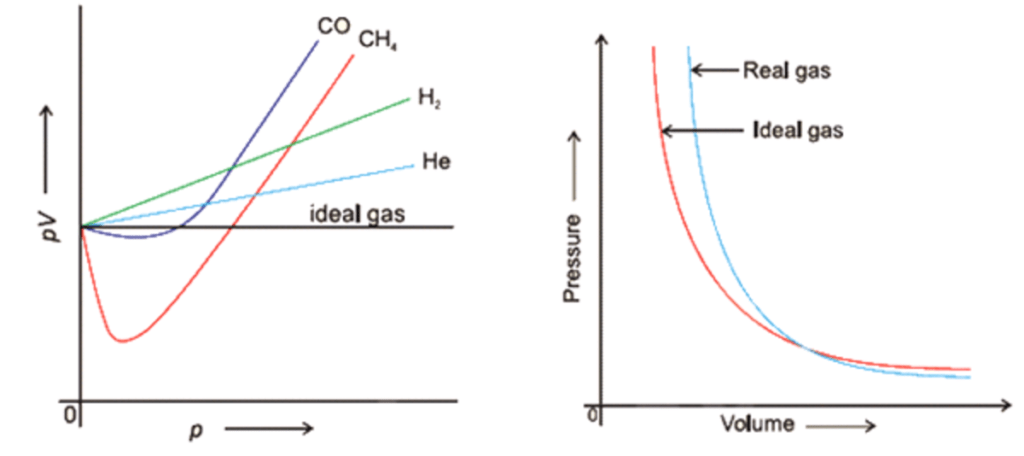A plot of pV vs p for real gas and ideal gas

At high pressure and low temperature, the gases deviate considerably from the ideal behaviour, we can express in terms of compressibility factor (Z), expressed as :

Z = pV/nRT

• case of ideal gas, pV = nRT Z = 1
• In the case of real gas, pV ≠ nRT, Z ≠ 1
• Negative deviation: In such case. Z < 1, gas is more compressible.
• Positive deviation: In such case. Z > 1 gas is less compressible.

### The factors affecting the deviation are

• Nature of the gas: In general, the most easily liquefiable and highly soluble gases show a larger deviation.
• Pressure: The deviation is more at high pressure. CO and N show negative deviation at low pressure and positive derivation at high pressure.
•  Temperature: The deviation is more at low temperature and H and He always shows positive deviations at O°C
Cause of deviation from the ideal behaviour:
It is due to two faulty assumptions of the kinetic theory of gases, particularly not valid at high pressure and low temperature.
• The volume occupied by the gas molecules is negligible as compared to the total volume of the gas.
• There are no attractive forces between the gas molecules.

## van der Waals’ Equation in States of matter

It states that after volume and pressure correction, van der Waals’ obtained the following equation for n moles of a gas.

Where,b = excluded volume or co-volume = 4 * actual volume of gas molecules.

a= magnitude of attractive forces between gas molecules.

The greater the value of ‘a’, the greater the strength of van der Waals’ forces and greater is the ease with which a gas can be liquefied.Units for van der Waals’ constant:
Pressure correction,

p = n2a/V2  or a = pV2/n2 = atm L2  mol-1

Volume correction,

V = nb  or b = V/n = L mol-1

Limitation of van der Waals’ equation:

• There is a specific range of temperature and pressure, to apply the equation.
• It deviates at very high pressure and very low temperature.

### Explanation of behaviour of Real Gases in States of matter

At very low pressures or at very high temperatures
pV = nRT
Gases behave like ideal gases

•  At moderate pressures, for 1 mole of a gas

pV/RT = 1 – a/RTV

Z = 1 – a/RTV

Z < 1

•  At high pressures, for 1 mole of a gas

PV/RT = 1 + Pb/RT

Z = 1 + pb/ RT

Z > 1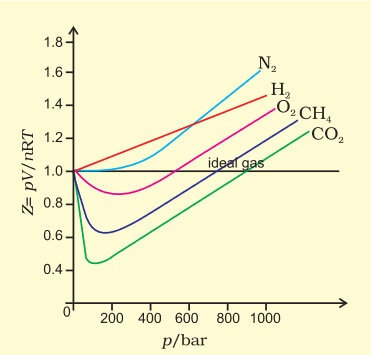#### Variation of compressibility factor for some gases:

Exceptional behaviour of H2 and He:

• This is due to the fact that intermolecular forces of attraction in them are negligible.
•  Value of ‘a’ is very small and thus ‘a/V2‘ is negligible

p (V – b) = RT

pV = RT + Pb

PV / RT = 1 + Pb/ RT

Z > 1 ( always, no dip in the curve of Z vs p).

## Liquefaction of Gases and Critical Points in States of matter

• The phenomenon of conversion of a gas into liquid is known as liquefaction.
• The liquefaction of gas takes place when the intermolecular forces of attraction become so high that exist in the liquid. A gas can be liquefied by

(i) increasing pressure.
(ii) decreasing temperature.

• Critical temperature (Tc):  It may be call as the temperature above which no gas can be liquefied. The critical temperature of CO2 is 31.1° C.
Critical temperature (Tc ) of some gases,
He (5.4), H2 (33.2), N2(126.0), CO(134.4), O2 (154.3), CO2 (3041), NH4 (405.5).
Tc = 8a/27Rb
• Critical pressure (Pc ): At the critical temperature, the pressure need to liquefy a gas is known as critical pressure.
Pc = a/27b2
• Critical volume (Vc): The volume occupied by one mole of a gas at critical temperature and critical pressure is known as critical volume.
Vc = 3b

Boyle’s temperature(Tb): Temperature at which a real gas exhibits ideal behaviour for a considerable range of pressure is
Tb = a/bR
Andrew’s Isotherms:  Isotherms of CO2 at different temperaturesDifference between vapour and gas:

• Above the critical temperature, CO2 is a gas and below a critical temperature, it is vapour .
• Also, vapour can be liquified by compression alone while a gas must be first cooled to a temperature below its critical temperature and then compressed.

## The Liquid State of Matter: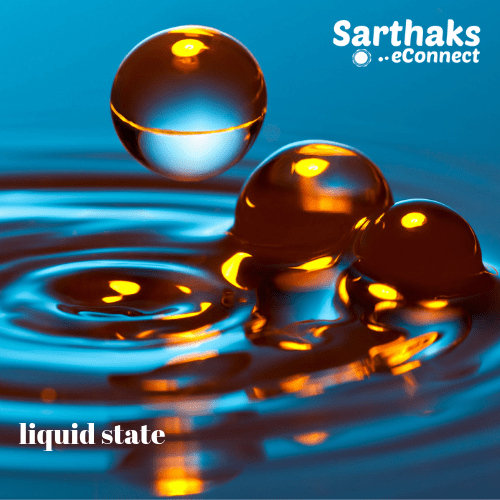•  If a substance is having a melting point below room temperature and a boiling point above room temperature, the substance is known as a liquid.
• In liquid, the matter has a definite shape and molecular motion is in between solids and gases.

### Properties of Liquids in States of Matter :

• Vapour pressure: The pressure exerted by the vapours above the liquid surface when these are in equilibrium with the liquid at a given temperature is known as the vapour pressure of a liquid.
• The vapour pressure of a liquid depends on :
• Nature of liquid
• Temperature: Vapour pressure increases with increasing temperature.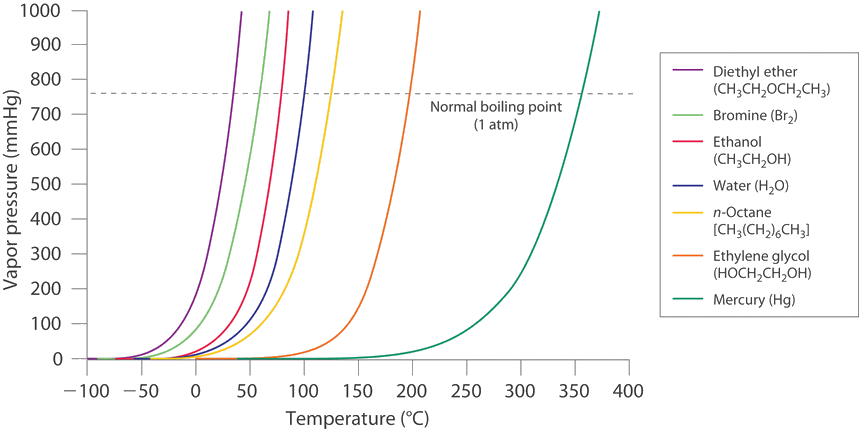•  Boiling point: The temperature at which vapour pressure of liquids becomes equal to the atmospheric pressure, is the boiling point.
• At 1 atm pressure, the boiling point is known as normal boiling point.
• At 1 bar pressure, the boiling point is known as standard boiling point. Boiling point varies linearly with external pressure.
• Surface tension: It is the force acting per unit length perpendicular to the imaginary line drawn on the surface of a liquid. It is symbol  γ (gamma);

SI unit: Nm-1

Dimensions: kgs-2

• The magnitude of surface tension of a liquid depends on the attractive forces between the molecules.
• We measure it with the help of an apparatus,  stalagmometer.
• Surface tension decreases as the temperature increases.
•  Rise or fall of liquid in a capillary tube is due to surface tension.
• Viscosity: Viscosity is a measure of resistance to flow which arises due to friction between layers of fluid.
• When there is a regular gradation of velocity, in passing from one layer to the next, it is laminar flow.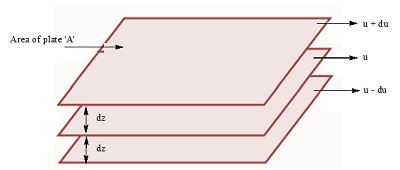Where

F = forces required to maintain the ow of layers.

A = area of contact

dv/ dz = velocity gradient; (the change in velocity with distance.)

‘η’ is proportionality constant and is called the coefficient of viscosity.
Viscosity is the force when the velocity gradient is unity and the area of contact is a unit area.
CGS unit of coefficient of viscosity is poise
S.I. unit of coefficient of viscosity is Nsm-2

## Important Questions With Answer from states of matter:

1. A gas deviates from ideal behaviour  at high pressure because of its molecules:
(a)  have kinetic energy                  (b) are bound by covalent bonds
(c)  attract one another                   (d) show the Tyndall effect

2. Which pair of gases diffuse with the same rate at the same temperature and pressure?
(a)  CO and NO                                   (b) NO2 and CO2
(c)   NH3 and PH3                                     (d) NO and C2H6

3.The term that corrects for the attractive forces present in a real gas in the van der Waals equation
is :
(a) nb                                                       (b) an2 /v2

(c)-an2/v2                                                 (d) – nb

4.  Most probable speed, average speed and rms speed are related as:
(a) 1 : 1.224 : 1.128                                      (b) 1.128 : 1 : 1.224
(c)  1 : 1.128 : 1.224                                     (d) 1.224 : 1.128 : 1

5. A sample of gas occupies 100 mL at 27 C and 740 mm pressure. When its volume is change to
80 mL at 740 mm pressure, the temperature of the gas will be
(a) 21.6°C                                                        (b) 240° C
(c) – 33°C                                                         (d) 89.5°C

ANS:

Since, Pressure is same
According to Charles law
V1/T1 = V2/T2  (T1 = 27 + 273 = 300 K)
100/300 = 80 /T2
T2 = 240 K = 240 – 273 = –33°C.

1.Komal Singh August 31, 2019